# Rotation of colorbar tick labels in Matplotlib

• Last Updated : 24 Jan, 2021

Colorbar is an axis that indicates the mapping of data values to the colors used in plot. The colorbar() function in pyplot module of matplotlib adds a colorbar to a plot indicating the color scale.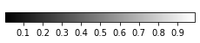Typical Colorbar

Sometimes it is desirable to rotate the ticklabels for better visualization and understanding. To change the rotation of colorbar ticklabels desired angle of rotation is provided in:

Attention geek! Strengthen your foundations with the Python Programming Foundation Course and learn the basics.

To begin with, your interview preparations Enhance your Data Structures concepts with the Python DS Course. And to begin with your Machine Learning Journey, join the Machine Learning - Basic Level Course

• cbar.ax.set_xticklabels, if colorbar orientation is horizontal
• cbar.ax.set_yticklabels, if colorbar orientation is vertical

Positive value of angle corresponds to counterclockwise rotation, while negative value corresponds to clockwise rotation. Also, we can use “vertical” and “horizontal” values for rotation instead of  numeric value of angle. These are equivalent to 0° and +90° respectively.

#### Steps to rotate colorbar ticklabels :

1. Plot a figure
2. Plot corresponding colorbar
3. Provide ticks and ticklabels
4. Set rotation of ticklabels to desired angle

Example 1: Following program demonstrates horizontal color bar with 45 degrees rotation of colorbar ticklabels.

## Python3

 `# Import libraries``import` `matplotlib.pyplot as plt``import` `numpy as np`` ` `# Plot image``a ``=` `np.random.random((``10``, ``10``))``plt.imshow(a, cmap``=``'gray'``)`` ` `# Plot horizontal colorbar``cbar ``=` `plt.colorbar(``    ``orientation``=``"horizontal"``, fraction``=``0.050``)`` ` `# Set ticklabels``labels ``=` `[``0``, ``0.1``, ``0.2``, ``0.3``, ``0.4``, ``0.5``, ``0.6``,``          ``0.7``, ``0.8``, ``0.9``, ``1``]``cbar.set_ticks(labels)`` ` `# Rotate colorbar ticklabels by 45 degrees``# anticlockwise``cbar.ax.set_xticklabels(labels, rotation``=``45``)`` ` `plt.show()`

Output: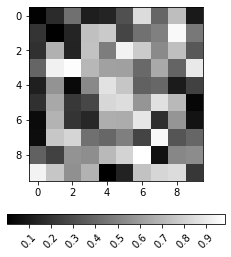Example 2: Following program demonstrates horizontal color bar with -45 degrees rotation of colorbar ticklabels.

## Python3

 `# Import libraries``import` `matplotlib.pyplot as plt``import` `numpy as np`` ` `# Plot image``a ``=` `np.random.random((``10``, ``10``))``plt.imshow(a, cmap``=``'gray'``)`` ` `# Plot horizontal colorbar``cbar ``=` `plt.colorbar(``    ``orientation``=``"horizontal"``, fraction``=``0.050``)`` ` `# Set ticklabels``labels ``=` `[``0``, ``0.1``, ``0.2``, ``0.3``, ``0.4``, ``0.5``, ``0.6``,``          ``0.7``, ``0.8``, ``0.9``, ``1``]``cbar.set_ticks(labels)`` ` `# Rotate colorbar ticklabels by 45 degrees clockwise``cbar.ax.set_xticklabels(labels, rotation``=``-``45``)`` ` `plt.show()`

Output: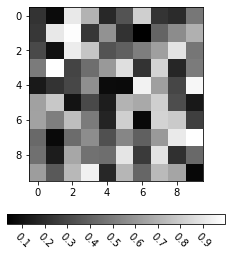Example 3: Following program demonstrates vertical color bar with 30 degrees rotation of colorbar ticklabels.

## Python3

 `# Import libraries``import` `matplotlib.pyplot as plt``import` `numpy as np`` ` `# Plot image``a ``=` `np.random.random((``10``, ``10``))``plt.imshow(a, cmap``=``'gray'``)`` ` `# Plot vertical colorbar``cbar ``=` `plt.colorbar(``    ``orientation``=``"vertical"``, fraction``=``0.050``)`` ` `# Set ticklabels``labels ``=` `[``0``, ``0.1``, ``0.2``, ``0.3``, ``0.4``, ``0.5``, ``0.6``,``          ``0.7``, ``0.8``, ``0.9``, ``1``]``cbar.set_ticks(labels)`` ` `# Rotate colorbar ticklabels by 30 degrees``# anticlockwise``cbar.ax.set_yticklabels(labels, rotation``=``30``)`` ` `plt.show()`

Output:Example 4: Following program demonstrates vertical color bar with -30 degrees rotation of colorbar ticklabels.

## Python3

 `# Import libraries``import` `matplotlib.pyplot as plt``import` `numpy as np`` ` `# Plot image``a ``=` `np.random.random((``10``, ``10``))``plt.imshow(a, cmap``=``'gray'``)`` ` `# Plot vertical colorbar``cbar ``=` `plt.colorbar(``    ``orientation``=``"vertical"``, fraction``=``0.050``)`` ` `# Set ticklabels``labels ``=` `[``0``, ``0.1``, ``0.2``, ``0.3``, ``0.4``, ``0.5``, ``0.6``,``          ``0.7``, ``0.8``, ``0.9``, ``1``]``cbar.set_ticks(labels)`` ` `# Rotate colorbar ticklabels by 30 degrees clockwise``cbar.ax.set_yticklabels(labels, rotation``=``-``30``)`` ` `plt.show()`

Output: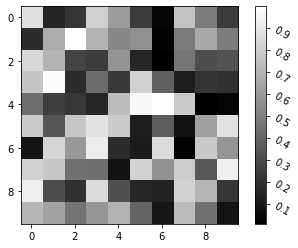Example 5: Following program demonstrates horizontal color bar with vertical rotation of colorbar ticklabels.

## Python3

 `# Import libraries``import` `matplotlib.pyplot as plt``import` `numpy as np`` ` `# Plot image``a ``=` `np.random.random((``10``, ``10``))``plt.imshow(a, cmap``=``'gray'``)`` ` `# Plot horizontal colorbar``cbar ``=` `plt.colorbar(``    ``orientation``=``"horizontal"``, fraction``=``0.050``)`` ` `# Set ticklabels``labels ``=` `[``0``, ``0.1``, ``0.2``, ``0.3``, ``0.4``, ``0.5``, ``0.6``,``          ``0.7``, ``0.8``, ``0.9``, ``1``]``cbar.set_ticks(labels)`` ` `# Rotate colorbar ticklabels by 90 degrees``# anticlockwise using "vertical" value``cbar.ax.set_xticklabels(labels,``                        ``rotation``=``"vertical"``)`` ` `plt.show()`

Output: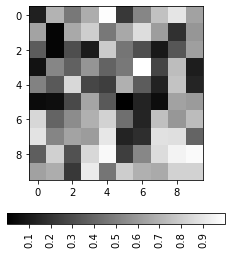Example 6: Following program demonstrates vertical color bar with 270 degrees rotation of colorbar ticklabels.

## Python3

 `# Import libraries``import` `matplotlib.pyplot as plt``import` `numpy as np`` ` `# Plot image``a ``=` `np.random.random((``10``, ``10``))``plt.imshow(a, cmap``=``'gray'``)`` ` `# Plot vertical colorbar``cbar ``=` `plt.colorbar(``    ``orientation``=``"vertical"``, fraction``=``0.050``)`` ` `# Set ticklabels``labels ``=` `[``0``, ``0.1``, ``0.2``, ``0.3``, ``0.4``, ``0.5``, ``0.6``,``          ``0.7``, ``0.8``, ``0.9``, ``1``]``cbar.set_ticks(labels)`` ` `# Rotate colorbar ticklabels by 270 degrees``# anticlockwise``cbar.ax.set_yticklabels(labels, rotation``=``270``)``plt.show()`

Output: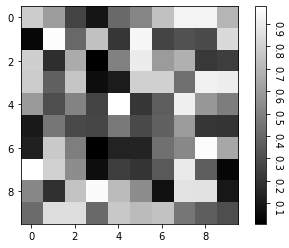My Personal Notes arrow_drop_up